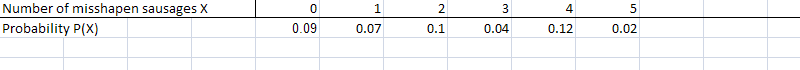# Make a Probability Distribution in Easy Steps + Video

Share on

Probability > Construct a probability distribution

## Make a probability distribution: Overview

A probability distribution is basically a chart of what the probability of an event happening is. It’s a way of quickly viewing the event, and the probability of that event. Probability distribution charts can get quite complex in statistics. For example, a normal distribution or t-distribution chart usually requires some form of technology (like Microsoft Excel or the TI-83 calculator) to create. However, you can construct a basic probability distribution showing events and probabilities in a few easy steps.

## Construct a probability distribution: Steps

Sample question: Construct a probability distribution for the following scenario: the probability of a sausage making machine producing 0, 1, 2, 3, 4, or 5 misshapen sausages per day are 0.09, 0.07, 0.1, 0.04,0.12, and 0.02.

• Step 1: Write down the number of widgets (things, items, products or other named thing) given on one horizontal line. In this case, the widgets in this question are the “misshapen sausages”.
• Step 2: Directly underneath the first line, write the probability of the event happening.
• The probability of the sausage machine producing 0 misshapen sausages a day is 0.09. Write “0.09” directly under “0”.
• The probability of the sausage machine producing 1 misshapen sausages a day is 0.07. Write “0.07” directly under “1”.
• The probability of the sausage machine producing 2 misshapen sausages a day is 0.1. Write “0.1” directly under “2”.
• The probability of the sausage machine producing 3 misshapen sausages a day is 0.04. Write “0.04” directly under “3”.
• The probability of the sausage machine producing 4 misshapen sausages a day is 0.12. Write “0.12” directly under “4”.
• The probability of the sausage machine producing 5 misshapen sausages a day is 0.02. Write “0.02” directly under “5”.That’s how to Construct a probability distribution!

Check out our YouTube Channel for hundreds more statistics how to videos!

CITE THIS AS:
Stephanie Glen. "Make a Probability Distribution in Easy Steps + Video" From StatisticsHowTo.com: Elementary Statistics for the rest of us! https://www.statisticshowto.com/how-to-construct-a-probability-distribution/
---------------------------------------------------------------------------Need help with a homework or test question? With Chegg Study, you can get step-by-step solutions to your questions from an expert in the field. Your first 30 minutes with a Chegg tutor is free!